Documentation

## Portfolio Optimization with Semicontinuous and Cardinality Constraints

This example shows how to use a `Portfolio` object to directly handle semicontinuous and cardinality constraints when performing portfolio optimization. Portfolio optimization finds the asset allocation that maximizes the return or minimizes the risk, subject to a set of investment constraints. The `Portfolio` class in Financial Toolbox™ is designed and implemented based on the Markowitz Mean-Variance Optimization framework. The Mean-Variance Optimization framework handles problems where the return is the expected portfolio return, and the risk is the variance of portfolio returns. Using the `Portfolio` class, you can minimize the risk on the efficient frontier (EF), maximize the return on the EF, maximize the return for a given risk, and minimize the risk for a given return. You can also use `PortfolioCVaR` or `PortfolioMAD` classes in Financial Toolbox™ to specify semicontinuous and cardinality constraints. Such optimization problems integrate with constraints such as group, linear inequality, turnover, and tracking error constraints. These constraints are formulated as nonlinear programming (NLP) problems with continuous variables represented as the asset weights ${x}_{i}$.

Semicontinuous and cardinality constraints are two other common categories of portfolio constraints that are formulated mathematically by adding the binary variables ${\mathit{v}}_{\mathit{i}}$.

• A semicontinuous constraint confines the allocation of an asset. For example, you can use this constraint to confine the allocated weight of an allocated asset to between 5% and 50%. By using this constraint, you can avoid very small or large positions to minimize the churns and operational costs. To mathematically formulate this type of constraint, a binary variable ${v}_{i}$ is needed, where ${\mathit{v}}_{\mathit{i}}$ is `0` or `1`. The value `0` indicates that asset i is not allocated and the value `1` indicates that asset i is allocated. The mathematical form is${\text{\hspace{0.17em}}\mathrm{lb}*{\mathit{v}}_{\mathit{i}}\le \mathit{x}}_{\mathit{i}}\le \mathrm{ub}*{\mathit{v}}_{\mathit{i}}$, where ${\mathit{v}}_{\mathit{i}}$ is `0` or `1`. Specify this type of constraint as a `'Conditional'` `BoundType` in the `Portfolio` class using the `setBounds` function.

• A cardinality constraint limits the number of assets in the optimal allocation, For example, for a portfolio with a universe of 100 assets, you can specify an optimal portfolio allocation between 20 and 40 assets. This capability helps limit the number of positions, and thus reduce operational costs. To mathematically formulate this type of constraint, binary variables represented as ${v}_{i}$ are needed, where ${\mathit{v}}_{\mathit{i}}$ is `0` or `1`. The value `0` indicates that asset i is not allocated and the value `1` indicates that asset i is allocated. The mathematical form is $\mathrm{MinNumAssets}\le {\sum }_{1}^{\mathrm{NumAssets}}{\mathit{v}}_{\mathit{i}}\le \mathrm{MaxNumAssets}$, where ${\mathit{v}}_{\mathit{i}}$ is `0` or `1`. Specify this type of constraint by setting the `'MinNumAssets'` and `'MaxNumAssets'`constraints in the `Portfolio` class using the `setMinMaxNumAssets` function.

For more information on semicontinuous and cardinality constraints, see Algorithms.

When semicontinuous and cardinality constraints are used for portfolio optimization, this leads to mixed integer nonlinear programming problems (MINLP). The `Portfolio` class allows you to configure these two constraints, specifically, semicontinuous constraints using `setBounds` with `'Conditional'` `BoundType`, and cardinality constraints using `setMinMaxNumAssets`. The `Portfolio` class automatically formulates the mathematical problems and validates the specified constraints. The `Portfolio` class also provides built-in MINLP solvers and flexible solver options for you to tune the solver performance using the `setSolverMINLP` function.

This example demonstrates a `Portfolio` object with semicontinuous and cardinality constraints and uses the `BlueChipStockMoments` dataset, which has a universe of 30 assets.

```load BlueChipStockMoments numAssets = numel(AssetList)```
```numAssets = 30 ```

### Limit the Minimum Weight for Each Allocated Asset

Create a fully invested portfolio with only long positions: ${\mathit{x}}_{\mathit{i}}\ge 0\text{\hspace{0.17em}\hspace{0.17em}}\mathrm{and}\text{\hspace{0.17em}}\mathrm{sum}\left({\mathit{x}}_{\mathit{i}}\right)=1$. These are configured with `setDefaultConstraints`.

```p = Portfolio('AssetList', AssetList,'AssetCovar', AssetCovar, 'AssetMean', AssetMean); p = setDefaultConstraints(p);```

Suppose that you want to avoid very small positions to minimize the churn and operational costs. Add another constraint to confine the allocated positions to be no less than 5%, by setting the constraints ${\mathit{x}}_{\mathit{i}}=0\text{\hspace{0.17em}}\mathrm{or}\text{\hspace{0.17em}}{\mathit{x}}_{\mathit{i}}\ge 0.05$ using `setBounds` with a `'Conditional'` `BoundType`.

`pWithMinWeight = setBounds(p, 0.05, 'BoundType', 'Conditional');`

Plot the efficient frontiers for both `Portfolio` objects.

```wgt = estimateFrontier(p); wgtWithMinWeight = estimateFrontier(pWithMinWeight); figure(1); plotFrontier(p, wgt); hold on; plotFrontier(pWithMinWeight, wgtWithMinWeight); hold off; legend('Baseline portfolio', 'With minWeight constraint', 'location', 'best');```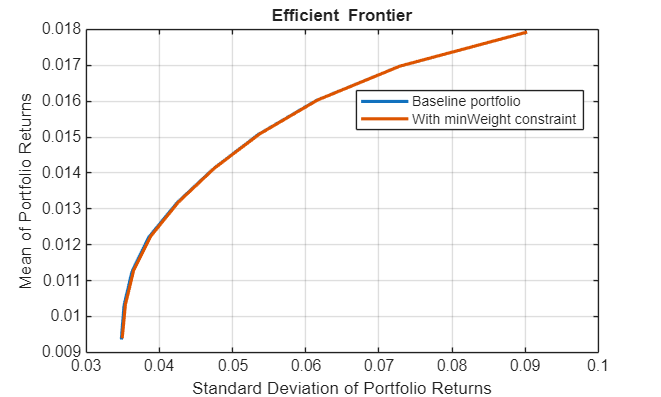The figure shows that the two `Portfolio` objects have almost identical efficient frontiers. However, the one with the minimum weight requirement is more practical, since it prevents the close-to-zero positions.

Check the optimal weights for the portfolio with default constraints to see how many assets are below the 5% limit for each optimal allocation.

```toler = eps; sum(wgt>toler & wgt<0.05)```
```ans = 1×10 5 7 5 4 2 3 4 2 0 0 ```

Use `estimateFrontierByReturn` to investigate the portfolio compositions for a target return on the frontier for both cases.

```targetRetn = 0.011; pwgt = estimateFrontierByReturn(p, targetRetn); pwgtWithMinWeight = estimateFrontierByReturn(pWithMinWeight, targetRetn);```

Plot the composition of the two `Portfolio` objects for the universe of 30 assets.

```figure(2); barh([pwgt, pwgtWithMinWeight]); grid on xlabel('Proportion of Investment') yticks(1:p.NumAssets); yticklabels(p.AssetList); title('Asset Allocation'); legend('Without min weight limit', 'With min weight limit', 'location', 'best');```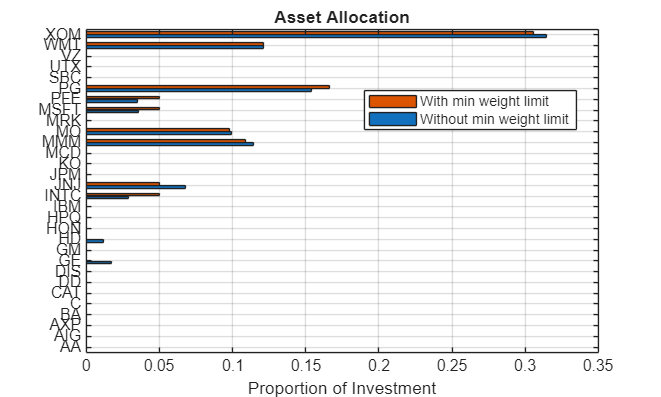Show only the allocated assets.

```idx = (pwgt>toler) | (pwgtWithMinWeight>toler); barh([pwgt(idx), pwgtWithMinWeight(idx)]); grid on xlabel('Proportion of Investment') yticks(1:sum(idx)); yticklabels(p.AssetList(idx)); title('Asset Allocation'); legend('Without min weight limit', 'With min weight limit', 'location', 'best');```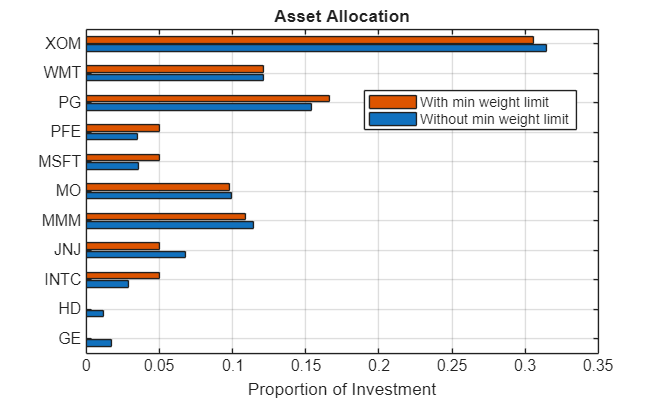### Limit the Maximum Number of Assets to Allocate

Use `setMinMaxNumAssets` to set the maximum number of allocated assets for the `Portfolio` object. Suppose that you want no more than eight assets invested in the optimal portfolio. To do this with a `Portfolio` object, use `setMinMaxNumAssets`.

```pWithMaxNumAssets = setMinMaxNumAssets(p, [], 8); wgt = estimateFrontier(p); wgtWithMaxNumAssets = estimateFrontier(pWithMaxNumAssets); plotFrontier(p, wgt); hold on; plotFrontier(pWithMaxNumAssets, wgtWithMaxNumAssets); hold off; legend('Baseline portfolio', 'With MaxNumAssets constraint', 'location', 'best');```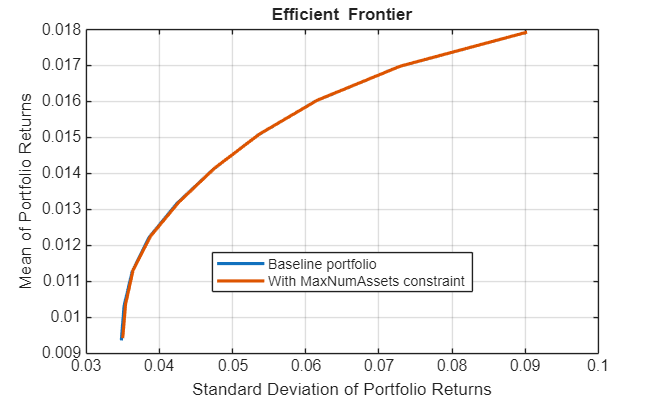Use `estimateFrontierByReturn` to find the allocation that minimizes the risk on the frontier for the given target return.

`pwgtWithMaxNum = estimateFrontierByReturn(pWithMaxNumAssets, targetRetn);`

Plot the composition of the two `Portfolio` objects for the universe of 30 assets.

```idx = (pwgt>toler) | (pwgtWithMaxNum>toler); barh([pwgt(idx), pwgtWithMaxNum(idx)]); grid on xlabel('Proportion of Investment') yticks(1:sum(idx)); yticklabels(p.AssetList(idx)); title('Asset Allocation'); legend('Baseline portfolio', 'With MaxNumAssets constraint', 'location', 'best');```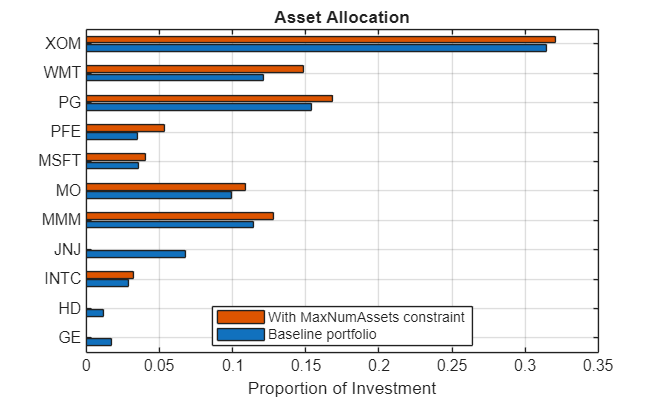`sum(abs(pwgt)>toler)`
```ans = 11 ```

Count the total number of allocated assets to verify that only eight assets at most are allocated.

`sum(abs(pwgtWithMaxNum)>toler)`
```ans = 8 ```

### Limit the Minimum and Maximum Number of Assets to Allocate

Suppose that you want to set both the lower and upper bounds for the number of assets to allocate in a portfolio, given the universe of assets. Use `setBounds` to specify the allowed number of assets to allocate as from 5 through 10, and the allocated weight as no less than 5%.

```p1 = setMinMaxNumAssets(p, 5, 10); p1 = setBounds(p1, 0.05, 'BoundType', 'conditional'); ```

If an asset is allocated, it is necessary to clearly define the minimum weight requirement for that asset. This is done using `setBounds` with a `'Conditional'` `BoundType`. Otherwise, the optimizer cannot evaluate which assets are allocated and cannot formulate the `MinNumAssets` constraint. For more details, see Conditional Bounds with LowerBound Defined as Empty or Zero.

Plot the efficient frontier to compare this portfolio to the baseline portfolio, which has only default constraints.

```wgt = estimateFrontier(p); wgt1 = estimateFrontier(p1); plotFrontier(p, wgt); hold on; plotFrontier(p1, wgt1); hold off; legend('Baseline portfolio', 'With MaxNumAssets constraint', 'location', 'best');```### Asset Allocation for an Equal-Weighted Portfolio

Create an equal-weighted portfolio using both `setBounds` and `setMinMaxNumAssets` functions.

```numAssetsAllocated = 8; weight= 1/numAssetsAllocated; p2 = setBounds(p, weight, weight, 'BoundType', 'conditional'); p2 = setMinMaxNumAssets(p2, numAssetsAllocated, numAssetsAllocated); ```

When any one, or any combination of `'Conditional'` `BoundType`, `MinNumAssets`, or `MaxNumAssets` are active, the optimization problem is formulated as a mixed integer nonlinear programming (MINLP) problem. The `Portfolio` class automatically constructs the MINLP problem based on the specified constraints.

When working with a `Portfolio` object, you can select one of three solvers using the `setSolverMINLP` function. In this example, instead of using default MINLP solver options, customize the solver options to help with a convergence issue. Use a large number (`50`) for `'MaxIterationsInactiveCut'` with `setSolverMINLP`, instead of the default value of `30` for '`MaxIterationsInactiveCut'`. The value `50` works well in finding the efficient frontier of optimal asset allocation.

`p2 = setSolverMINLP(p2, 'OuterApproximation', 'MaxIterationsInactiveCut', 50);`

Plot the efficient frontiers for the baseline and equal-weighted portfolios.

```wgt = estimateFrontier(p); wgt2 = estimateFrontier(p2); plotFrontier(p, wgt); hold on; plotFrontier(p2, wgt2); hold off; legend('Baseline portfolio', 'Equal Weighted portfolio', 'location', 'best');```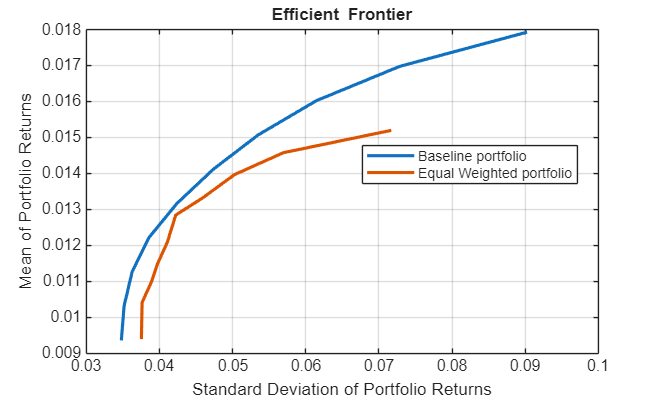Use `estimateFrontierByRisk` to optimize for a specific risk level, in this case `.05`, to determine what allocation maximizes the portfolio return.

```targetRisk = 0.05; pwgt = estimateFrontierByRisk(p, targetRisk); pwgt2 = estimateFrontierByRisk(p2, targetRisk); idx = (pwgt>toler) | (pwgt2>toler); barh([pwgt(idx), pwgt2(idx)]); grid on xlabel('Proportion of investment') yticks(1:sum(idx)); yticklabels(p.AssetList(idx)); title('Asset Allocation'); legend('Baseline portfolio', 'Equal weighted portfolio', 'location', 'best');```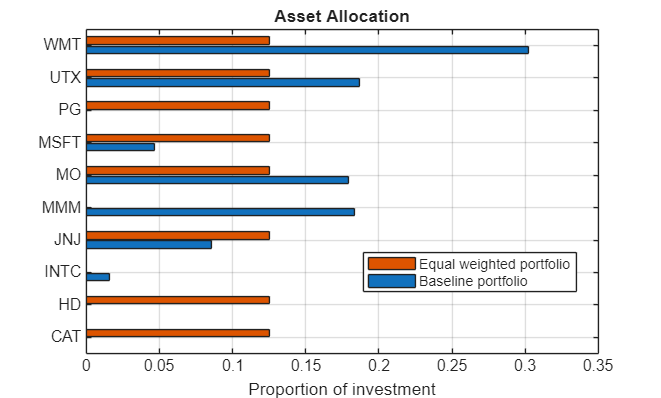### Use `'Conditional'``BoundType`, `MinNumAssets`, and `MaxNumAssets` Constraints with Other Constraints

You can define other constraints for a `Portfolio` object using the `set` functions. These other constraints for a `Portfolio` object, such as group, linear inequality, turnover, and tracking error can be used together with the `'Conditional'` `BoundType`, `'MinNumAssets'`, and `'MaxNumAssets'` constraints. For example, specify a tracking error constraint using `setTrackingError`.

```ii = [15, 16, 20, 21, 23, 25, 27, 29, 30]; % indexes of assets to include in tracking portfolio trackingPort(ii) = 1/numel(ii); q = setTrackingError(p, 0.5, trackingPort);```

Then use `setMinMaxNumAssets` to add a constraint to limit maximum number of assets to invest.

`q = setMinMaxNumAssets(q, [], 8);`

On top of these previously specified constraints, use `setBounds` to add a constraint to limit the weight for the allocated assets. You can use constraints with mixed `BoundType` values, where `'Simple'` means $\mathrm{lb}\le {\mathit{x}}_{\mathit{i}}\le \mathrm{ub}\text{\hspace{0.17em}}$and `'Conditional'` means ${\mathit{x}}_{\mathit{i}}=0\text{\hspace{0.17em}}\mathrm{or}\text{\hspace{0.17em}}{\text{\hspace{0.17em}}\mathrm{lb}\le \mathit{x}}_{\mathit{i}}\le \mathrm{ub}$.

Allow the assets in `trackingPort` to have the `BoundType` value `'Conditional'` in the optimum allocation.

```lb = zeros(q.NumAssets, 1); ub = zeros(q.NumAssets, 1)*0.5; lb(ii) = 0.1; ub(ii) = 0.3; boundType = repmat("simple",q.NumAssets,1); boundType(ii) = "conditional"; q = setBounds(q, lb, ub, 'BoundType',boundType);```

Plot the efficient frontier:

`plotFrontier(q);`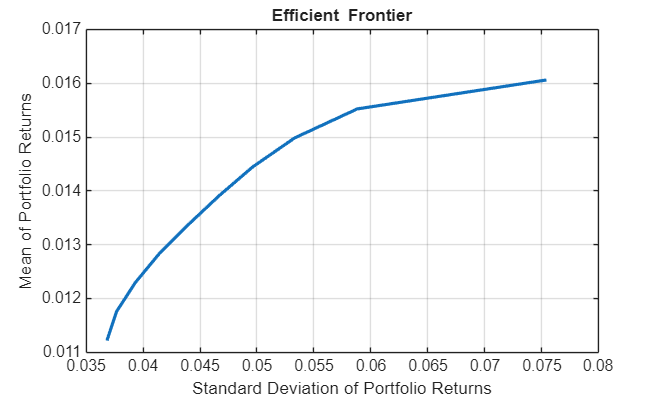Use `estimateFrontierByReturn` to find the allocation that minimizes the risk for a given return at `0.125`.

```targetRetn = 0.0125; pwgt = estimateFrontierByReturn(q, targetRetn);```

Show the allocation of assets by weight.

```idx = abs(pwgt)>eps; assetnames = q.AssetList'; Asset = assetnames(idx); Weight = pwgt(idx); resultAlloc = table(Asset, Weight)```
```resultAlloc=7×2 table Asset Weight ________ _______ {'JNJ' } 0.1 {'MMM' } 0.19503 {'MO' } 0.1485 {'MSFT'} 0.1 {'PG' } 0.1 {'WMT' } 0.2212 {'XOM' } 0.13527 ```